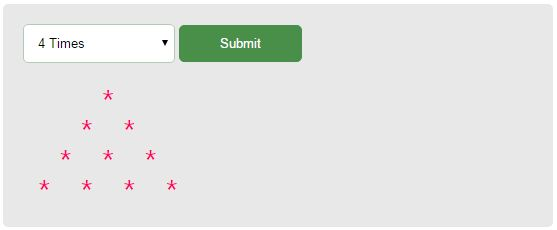# PHP Loops

Loops are used to run the same lines of code multiple times. If we want to execute the same code multiple times then we can put it into a loop block to avoid code repetition. The loop structure includes three sections. These are,

• Initialization – To initialize the first iteration index of the loop.
• Conditional execution – For each iteration, it checks whether the specified condition is satisfied. If so, then only the code will be executed.
• Incrementation/decrementation – After processing each iteration, the loop index should be incremented or decremented to process the next iteration. This will be continued till the loop condition is satisfied.

PHP supports four types of loop statements. These are, while, do…while, for and foreach. In this tutorial, we are going to see about each of these types of PHP loop statements with an example. I have added a demo with a nested for loop to print the star triangle.

View Demo

## while

The following example code shows a while loop with initialization, conditional expression, and incrementation. In this example, I initialize the loop index \$i to 1.

The while loop will echo the loop index.  Then, the index will be checked with the while condition to process the code within the loop block. The loop will print the index value and increment it for each iteration. This will be continued till the while loop condition is satisfied.

``````<?php
\$i = 1;
while (\$i <= 3) {
echo \$i;
\$i ++;
}
?>
``````

## do..while

do..while is the same as while loop. It has the same three parts initialization, conditional execution, and incrementation/decrementation.

The difference is that it will check the condition after processing an iteration and before going to the next iteration. So, in do..while there is an assured guarantee for the execution of the first iteration. I changed the while loop example code with do…while. The code is,

``````<?php
\$i = 1;
do {
echo \$i;
\$i ++;
} while ((\$i > 1) && (\$i <= 3));
?>
``````

## for

The for loop is so easy to understand and it has a very simple structure to use it in programming. It has the initialization, conditional statement and increments/decrements in a single line as shown in the below syntax.

``````for(initialization; conditional statement; increments (or) decrements) {
//set of expressions to be execute recursively
}
``````

The while loop example is converted to the for loop to print the loop index for the three iterations.

``````<?php
for (\$i = 1; \$i <= 3; \$i ++) {
echo \$i;
}
?>
``````

## foreach

The foreach loop is used to iterate the array variables to process the array keys and values. The following code snippet shows the syntax of the foreach statement with two types of usage.

The first type is used to move the array pointer one step inner level or multidimensional array. The second line type is used to iterate an array and process with its key and value pairs for each iteration.

``````<?php
foreach (\$array as \$value_array) {}
// (or)
foreach (\$array as \$key_array => \$value_array) {}
?>
``````

## OutputNote: Though PHP has various types of loop statements, the while loop usage is the optimistic way of programming. Because any type of loop structure will be changed to while at the time of compilation.